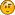###Author Topic: Angle Alignment  (Read 2582 times)

####gonso##### Angle Alignment
« on: December 20, 2008, 08:36:54 pm »
Good afternoon,

My approach is the common one, bring the objects to the origin, unrotate them in ZYX order, rotate in XYZ order and place them in their new position. Everything working fine.  I need to code a function:

public void align(Object3D obj, SimpleVector position, SimpleVector angles) and/or
public void align(Object3D obj, SimpleVector v1, SimpleVector v2)

That puts the object in the position and angles given (first version) or puts the object in v1 and facing the direction
of the vector that goes from v1 to v2.

and, finally:

public void lookAt(Object3D obj,SimpleVector point)

That rotates the object whose coordinates are wx,wy,wz and angles ax,ay,az so that it looks at the point. I have seen that there is an Align function for Object3D already, but, probably due to being a newbee, I am unable to obtain the local angles of an object once it has been rotated, just to store them for route logging, for example. Getting the position back is easy, the angles are not ¿Any idea?

In 2D this seems to be easy, just using atan2... but in 3D it is resulting hard for me. I would l ike to implement it with
moveTo, and Rotate or RotateX, RotateY RotateZ operands, avoiding low level matrix manipulation as the transformations are centered in a function that is automatically called before rendering, it is name update(Object3D ..). The current positions and angles of the object are stored for simplicity and named wx,wy,wz  and ax,ay,az for angles. There is a copy of the last know position and angles in owx,owy,owz and oax,oay,oaz (o stands for 'old')

Have read about angle - axis solution but my implementation is failing. Are quaternions the answer? Any help would be really appreciated.

Ramon Talavera
« Last Edit: December 20, 2008, 08:47:26 pm by gonso »

####paulscode

• double
••• Posts: 863##### Re: Angle Alignment
« Reply #1 on: December 21, 2008, 05:21:42 am »
I wasn't clear about the first method you mentioned, exactly what data is contained in SimpleVecor 'angles'?

Other than that, the following should work for the other two methods:

Code: [Select]
`public void align( Object3D object, SimpleVector position, SimpleVector target ){    SimpleVector translation = position.calcSub( obj.getTransformedCenter() );    object.translate( translation );    lookAt( object, target );}`
Code: [Select]
`public void lookAt( Object3D object, SimpleVector target ){    SimpleVector direction = new SimpleVector( target.calcSub( object.getTransformedCenter() ) ).normalize();    Matrix rotationMatrix = new Matrix( direction.getRotationMatrix() );    object.setRotationMatrix( rotationMatrix );}`

####paulscode

• double
••• Posts: 863##### Re: Angle Alignment
« Reply #2 on: December 21, 2008, 04:43:39 pm »
After re-reading your post, I understand now what you mean for the first method - using rotations around the X,Y,Z axises.  Another option would be to calculate an axis to rotate around.  A few weeks ago I had attempted to write a "lookAt" method like this using the law of cosines:

Code: [Select]
`//  initial direction:SimpleVector directionI = new SimpleVector( object.getZAxis() ).normalize();//  destination direction:SimpleVector directionD = new SimpleVector( target.getTransformedCenter().calcSub( object.getTransformedCenter() ) ).normalize();//  axis to rotate around:SimpleVector axis = new SimpleVector( directionI.calcCross( directionD ) ).normalize();//  ** PROBLEM AFTER THIS POINT **//  length of the triangle's third side:float distC = directionD.calcSub( directionI ).length();//  Angle to rotate:float angle = (float) Math.acos( (2.0f - (distC * distC)) / 2.0f );// performe the rotation:object.rotateAxis( axis, angle );`
As you can see, I ran into a brick wall on this due to the fact that the formula uses arc cosine, so the object can not rotate more than PI radians at a time.  If you know of a better formula, you might be able to get something like the above to work.
« Last Edit: December 21, 2008, 05:09:04 pm by paulscode »

####gonso##### Re: Angle Alignment
« Reply #3 on: December 21, 2008, 07:07:04 pm »
Yep, but the solution you gave me was using JPCT's lookAt function, and works , from that perspective my question would be:

If LookAt method is working (it does) the question is:

------------------------------------------------------------------------------------------------------------------------------------------
Basically there is a accessor method for an object's position (getPosition or getTransformedCenter) but no getAngles/getTransformedAngles...
How are object angles obtained after the rotation?

-------------------------------------------------------------------------------------------------------------------------------------------

Once lookAt method has been called , how could I get the resulting angles back? How are the local angles (relative to the object's central axis) obtained now?

public void align( Object3D object, SimpleVector position, SimpleVector target )
{
SimpleVector translation = position.calcSub( obj.getTransformedCenter() );

// Store current position and angles:
oldposition=object.getPosition();
oldangle=object.getLocalAngles(); <==========

object.translate( translation );
lookAt( object, target );

position=object.getPosition();
angle=object.getLocalAngles();  <================ HOW IS THIS DONE?}

I also tried with the acos approach and atan2 approach and had the same problems that you had.

Thanks for your quick reply.

« Last Edit: December 21, 2008, 08:16:16 pm by gonso »